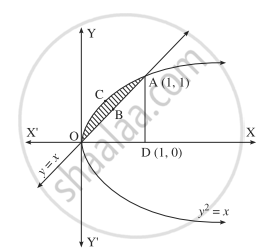# Solve the following : Find the area of the region bounded by the parabola y2 = x and the line y = x in the first quadrant. - Mathematics and Statistics

Sum

Solve the following :

Find the area of the region bounded by the parabola y2 = x and the line y = x in the first quadrant.

#### Solution

To obtain the points of intersection of the line and the parabola, we equate the values of x from both the equations.∴ y2 = y
∴ y2 – y = 0
∴ y(y – 1) = 0
∴ y = 0 or y = 1
When y = 0, x = 0
When y = 1, x = 1
∴ the points of intersection are O(0, 0) and A(1, 1). Required area:area of the region OCABO
= area of the region OCADO – area of the region OBADO
Now, area of the region OCADO
= area under the parabola y2 = x i.e. y = ± sqrt(x) (in the first quadrant) between x = 0 and x = 1

= int_0^1 sqrt(x)*dx

= [(x^(3/2))/(3/2)]_0^1

= (2)/(3) xx (1 - 0)

= (2)/(3)
= area under the line y = x between x  0 and x = 1

= int_0^1x*dx

=[x^2/2]_0^1

=(1)/(2) - 0

= (2)/(3)
∴ required area = (2)/(3) - (1)/(2)

= (1)/(6)"sq unit".

Concept: Area Bounded by the Curve, Axis and Line
Is there an error in this question or solution?
Chapter 5: Application of Definite Integration - Miscellaneous Exercise 5 [Page 190]

#### APPEARS IN

Balbharati Mathematics and Statistics 2 (Arts and Science) 12th Standard HSC Maharashtra State Board
Chapter 5 Application of Definite Integration
Miscellaneous Exercise 5 | Q 2.06 | Page 190
Share